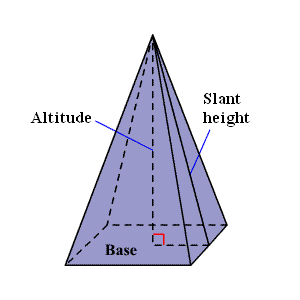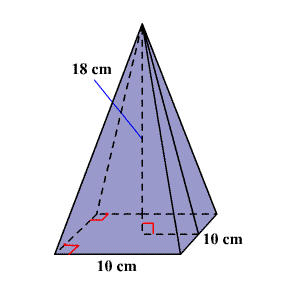# Volume of a Pyramid

A pyramid is a polyhedron with one base that is any polygon . Its other faces are triangles.The volume of a $3$ -dimensional solid is the amount of space it occupies.  Volume is measured in cubic units ( ${\text{in}}^{3},{\text{ft}}^{3},{\text{cm}}^{3},{\text{m}}^{3}$ , et cetera).  Be sure that all of the measurements are in the same unit before computing the volume.

The volume $V$ of a pyramid is one-third the area of the base $B$ times the height $h$ .

$V=\frac{1}{3}Bh$

Example:

Find the volume of a regular square pyramid with base sides $10$ cm and altitude $18$ cm.

Solution

Draw a figure.The formula for the volume of a pyramid is,

$V=\frac{1}{3}Bh$

Since the base of the pyramid is a square, the base area is ${10}^{2}$ or $100\text{\hspace{0.17em}}\text{\hspace{0.17em}}{\text{cm}}^{2}$ .

So, substitute $100$ for $B$ and $18$ for $h$ in the formula.

$\begin{array}{l}V=\frac{1}{3}\left(100\right)\left(18\right)\\ =600\end{array}$

Therefore, the volume of the square pyramid is $600\text{\hspace{0.17em}}\text{\hspace{0.17em}}{\text{cm}}^{3}$ .